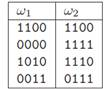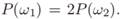# Consider the following patterns, each having four binary-valued attributes: Note especially that the first patterns in the two categories are the same. (a) Create by hand a binary classification tree for this data. Train your tree so that the leaf nodes have the lowest impurity possible. (b) Suppose it is known that during testing the prior probabilities of the two categories will not be equal, but instead   Modify your training method and use the above data to form a tree for this case.

Question
1 views

Consider the following patterns, each having four binary-valued attributes:Note especially that the first patterns in the two categories are the same.

(a) Create by hand a binary classification tree for this data. Train your tree so that the leaf nodes have the lowest impurity possible.

(b) Suppose it is known that during testing the prior probabilities of the two categories will not be equal, but insteadModify your training method and use the above data to form a tree for this case.

### This question hasn't been answered yet.